# Working With Negative Fractional Exponents (Math)Hello. In this mathematics post, I will go over dealing with negative fractional exponents. Negative exponents can be annoying to deal with along with fractional exponents that may appear in the form of square roots. This algebra concept is needed as a necessary skill for the algebra toolkit in pre-calculus, calculus.

It is assumed that the reader is comfortable with exponent laws, square roots and fractions.

The math text here is rendered with a lot of LaTeX (lay-tech) along with the use of QuickLatex.comPixabay Image Source

## Topics

• Negative Exponents Review
• Fractional Exponents Review
• Dealing With Negative Fractional Exponents
• Practice Problems With Solutions

## Negative Exponents Review

Do remember that with negative exponents you do not include negative numbers. The negative exponent actually represents taking the reciprocal (flipping the fraction).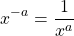and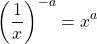where `a` is a non-zero number.

If you have a fraction with a negative exponent, you still flip the fraction and apply the (positive) exponent to the numerator and denominator.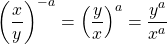The main reason why answers are written with positive exponents is because of styling and common acceptance.

Example One

Evaluate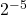.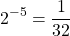Example Two

Rewrite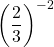into a fraction with positive exponents.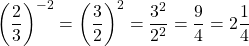Example Three

Evaluate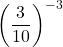.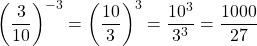Example Four

Rewrite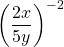into a fraction with positive exponents.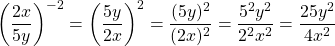Pixabay Image Source

## Fractional Exponents Review

It can take some time to get used to the idea of fractional exponents and working with them. A common example that I like to use when I help students with mathematics is the square root. The square root of `x` for example is actually `x` raised to the power of one half.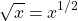The cubic root has an exponent of one third and can be negative.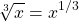In general, the n-th root can be expressed as 1 divided by n as an exponent. Define `n` as a whole number.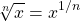Example 1a

Evaluating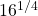gives 2 as `2 x 2 x 2 x 2 = 16`.

Example 1b

Evaluating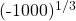gives -10 as `-10 x -10 x -10 = -1000`.

Everything so far in this section dealt with fractional exponents with a numerator of 1. What if we have fractional exponents with numerators greater than one? Let's see some examples.

Example 2a

What is the value of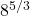?

When it comes to fractional exponents I like to work with the denominator part of the exponent and take the n-th root first. The third root of 8 is 2. From the two, apply the exponent of five to the two to obtain 32.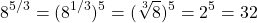I use the reverse procedure of the power to a power exponent law. Recall that we have: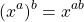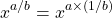Example 2b

Evaluate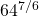.

You could evaluate 64 to the power of 7 first but you could end up with a really large number. Large numbers are annoying to deal with as you have to write more digits and press more buttons on a calculator.

The sixth root of 64 is evaluated first to obtain 2. Then evaluate 2 to the power of 7 which is equal to 128.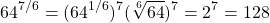Pixabay Image Source

## Dealing With Negative Fractional Exponents

So far we have dealt with negative exponents and fractional exponents separately. This section will put it all together.

I generally like to deal with the negative parts from the exponents then deal with the fractional exponents.

Example One

Evaluate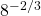.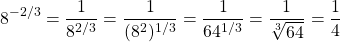Example Two

What is the numeric value of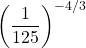?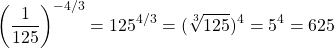Example Three

Simplify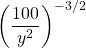.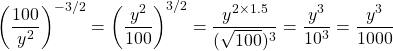Example Four

Simplifyand rewrite this with positive exponents.

With this one I simplify the stuff inside the bracket first. Then I apply the negative part of the exponent to obtain 1 divided by the product of x-cubed and y-squared.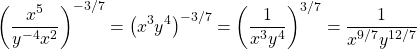Pixabay Image Source

## Practice Problems With Solutions

Here are some practice problems for building understanding and to improve algebra speed. Refer to the examples as a guide. For all the questions, simplify the following and express answers with positive exponents.

Question One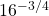Question Two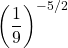Question Three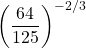Question Four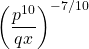Question Five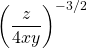Solutions To Practice Problems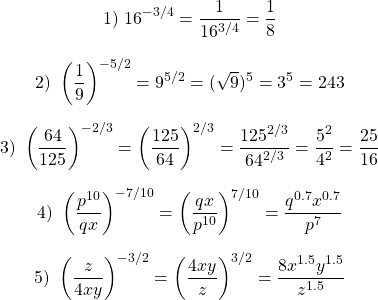Pixabay Image Source

Posted with STEMGeeks

0
0
0.000